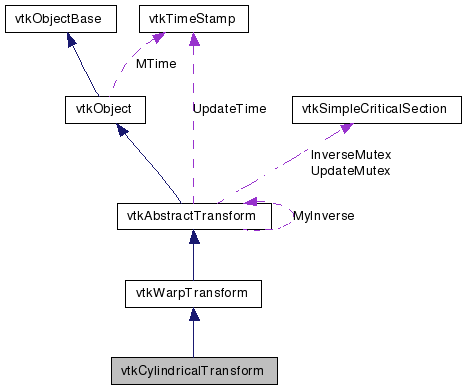# vtkCylindricalTransform Class Reference

`#include <vtkCylindricalTransform.h>`

Inheritance diagram for vtkCylindricalTransform:[legend]
Collaboration diagram for vtkCylindricalTransform:[legend]

## Detailed Description

cylindrical to rectangular coords and back

vtkCylindricalTransform will convert (r,theta,z) coordinates to (x,y,z) coordinates and back again. The angles are given in radians. By default, it converts cylindrical coordinates to rectangular, but GetInverse() returns a transform that will do the opposite. The equation that is used is x = r*cos(theta), y = r*sin(theta), z = z.

Warning:
This transform is not well behaved along the line x=y=0 (i.e. along the z-axis)
vtkSphericalTransform vtkGeneralTransform
Tests:
vtkCylindricalTransform (Tests)

Definition at line 38 of file vtkCylindricalTransform.h.

## Public Types

typedef vtkWarpTransform Superclass

## Public Member Functions

virtual const char * GetClassName ()
virtual int IsA (const char *type)
virtual void PrintSelf (ostream &os, vtkIndent indent)
vtkAbstractTransformMakeTransform ()

## Static Public Member Functions

static vtkCylindricalTransformNew ()
static int IsTypeOf (const char *type)
static vtkCylindricalTransformSafeDownCast (vtkObject *o)

## Protected Member Functions

vtkCylindricalTransform ()
~vtkCylindricalTransform ()
void InternalDeepCopy (vtkAbstractTransform *transform)
void ForwardTransformDerivative (const float in, float out, float derivative)
void ForwardTransformDerivative (const double in, double out, double derivative)
void InverseTransformPoint (const float in, float out)
void InverseTransformPoint (const double in, double out)
void InverseTransformDerivative (const float in, float out, float derivative)
void InverseTransformDerivative (const double in, double out, double derivative)
void ForwardTransformPoint (const float in, float out)
void ForwardTransformPoint (const double in, double out)

## Member Typedef Documentation

Reimplemented from vtkWarpTransform.

Definition at line 42 of file vtkCylindricalTransform.h.

## Constructor & Destructor Documentation

 vtkCylindricalTransform::vtkCylindricalTransform ( ) ` [protected]`

 vtkCylindricalTransform::~vtkCylindricalTransform ( ) ` [protected]`

## Member Function Documentation

 static vtkCylindricalTransform* vtkCylindricalTransform::New ( ) ` [static]`

Create an object with Debug turned off, modified time initialized to zero, and reference counting on.

Reimplemented from vtkObject.

 virtual const char* vtkCylindricalTransform::GetClassName ( ) ` [virtual]`

Reimplemented from vtkWarpTransform.

 static int vtkCylindricalTransform::IsTypeOf ( const char * name ) ` [static]`

Return 1 if this class type is the same type of (or a subclass of) the named class. Returns 0 otherwise. This method works in combination with vtkTypeRevisionMacro found in vtkSetGet.h.

Reimplemented from vtkWarpTransform.

 virtual int vtkCylindricalTransform::IsA ( const char * name ) ` [virtual]`

Return 1 if this class is the same type of (or a subclass of) the named class. Returns 0 otherwise. This method works in combination with vtkTypeRevisionMacro found in vtkSetGet.h.

Reimplemented from vtkWarpTransform.

 static vtkCylindricalTransform* vtkCylindricalTransform::SafeDownCast ( vtkObject * o ) ` [static]`

Reimplemented from vtkWarpTransform.

 virtual void vtkCylindricalTransform::PrintSelf ( ostream & os, vtkIndent indent ) ` [virtual]`

Methods invoked by print to print information about the object including superclasses. Typically not called by the user (use Print() instead) but used in the hierarchical print process to combine the output of several classes.

Reimplemented from vtkWarpTransform.

 vtkAbstractTransform* vtkCylindricalTransform::MakeTransform ( ) ` [virtual]`

Make another transform of the same type.

Implements vtkAbstractTransform.

 void vtkCylindricalTransform::InternalDeepCopy ( vtkAbstractTransform * transform ) ` [protected, virtual]`

Copy this transform from another of the same type.

Reimplemented from vtkAbstractTransform.

 void vtkCylindricalTransform::ForwardTransformPoint ( const float in, float out ) ` [protected, virtual]`

Internal functions for calculating the transformation.

Implements vtkWarpTransform.

 void vtkCylindricalTransform::ForwardTransformPoint ( const double in, double out ) ` [protected, virtual]`

Internal functions for calculating the transformation.

Implements vtkWarpTransform.

 void vtkCylindricalTransform::ForwardTransformDerivative ( const float in, float out, float derivative ) ` [protected, virtual]`

Calculate the forward transform as well as the derivative.

Implements vtkWarpTransform.

 void vtkCylindricalTransform::ForwardTransformDerivative ( const double in, double out, double derivative ) ` [protected, virtual]`

Calculate the forward transform as well as the derivative.

Implements vtkWarpTransform.

 void vtkCylindricalTransform::InverseTransformPoint ( const float in, float out ) ` [protected, virtual]`

If the InverseFlag is set to 1, then a call to InternalTransformPoint results in a call to InverseTransformPoint. The inverse transformation is calculated from using Newton's method.

Reimplemented from vtkWarpTransform.

 void vtkCylindricalTransform::InverseTransformPoint ( const double in, double out ) ` [protected, virtual]`

If the InverseFlag is set to 1, then a call to InternalTransformPoint results in a call to InverseTransformPoint. The inverse transformation is calculated from using Newton's method.

Reimplemented from vtkWarpTransform.

 void vtkCylindricalTransform::InverseTransformDerivative ( const float in, float out, float derivative ) ` [protected, virtual]`

Calculate the inverse transform as well as the derivative of the forward transform (that's correct: the derivative of the forward transform, not of the inverse transform)

Reimplemented from vtkWarpTransform.

 void vtkCylindricalTransform::InverseTransformDerivative ( const double in, double out, double derivative ) ` [protected, virtual]`

Calculate the inverse transform as well as the derivative of the forward transform (that's correct: the derivative of the forward transform, not of the inverse transform)

Reimplemented from vtkWarpTransform.

The documentation for this class was generated from the following file:

Generated on Wed Jun 3 18:58:56 2009 for VTK by1.5.6